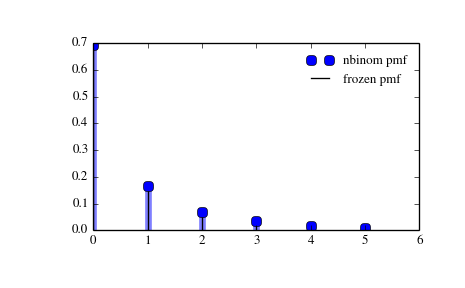# scipy.stats.nbinom¶

scipy.stats.nbinom = <scipy.stats._discrete_distns.nbinom_gen object at 0x2b45d2fe2b10>[source]

A negative binomial discrete random variable.

Discrete random variables are defined from a standard form and may require some shape parameters to complete its specification. Any optional keyword parameters can be passed to the methods of the RV object as given below:

Parameters: x : array_like quantiles q : array_like lower or upper tail probability n, p : array_like shape parameters loc : array_like, optional location parameter (default=0) size : int or tuple of ints, optional shape of random variates (default computed from input arguments ) moments : str, optional composed of letters [‘mvsk’] specifying which moments to compute where ‘m’ = mean, ‘v’ = variance, ‘s’ = (Fisher’s) skew and ‘k’ = (Fisher’s) kurtosis. (default=’mv’) Alternatively, the object may be called (as a function) to fix the shape and location parameters returning a “frozen” discrete RV object: rv = nbinom(n, p, loc=0) Frozen RV object with the same methods but holding the given shape and location fixed.

Notes

The probability mass function for nbinom is:

```nbinom.pmf(k) = choose(k+n-1, n-1) * p**n * (1-p)**k
```

for k >= 0.

nbinom takes n and p as shape parameters.

Examples

```>>> from scipy.stats import nbinom
>>> import matplotlib.pyplot as plt
>>> fig, ax = plt.subplots(1, 1)
```

Calculate a few first moments:

```>>> n, p = 0.4, 0.4
>>> mean, var, skew, kurt = nbinom.stats(n, p, moments='mvsk')
```

Display the probability mass function (pmf):

```>>> x = np.arange(nbinom.ppf(0.01, n, p),
...               nbinom.ppf(0.99, n, p))
>>> ax.plot(x, nbinom.pmf(x, n, p), 'bo', ms=8, label='nbinom pmf')
>>> ax.vlines(x, 0, nbinom.pmf(x, n, p), colors='b', lw=5, alpha=0.5)
```

Alternatively, freeze the distribution and display the frozen pmf:

```>>> rv = nbinom(n, p)
>>> ax.vlines(x, 0, rv.pmf(x), colors='k', linestyles='-', lw=1,
...         label='frozen pmf')
>>> ax.legend(loc='best', frameon=False)
>>> plt.show()
```Check accuracy of cdf and ppf:

```>>> prob = nbinom.cdf(x, n, p)
>>> np.allclose(x, nbinom.ppf(prob, n, p))
True
```

Generate random numbers:

```>>> r = nbinom.rvs(n, p, size=1000)
```

Methods

 rvs(n, p, loc=0, size=1) Random variates. pmf(x, n, p, loc=0) Probability mass function. logpmf(x, n, p, loc=0) Log of the probability mass function. cdf(x, n, p, loc=0) Cumulative density function. logcdf(x, n, p, loc=0) Log of the cumulative density function. sf(x, n, p, loc=0) Survival function (1-cdf — sometimes more accurate). logsf(x, n, p, loc=0) Log of the survival function. ppf(q, n, p, loc=0) Percent point function (inverse of cdf — percentiles). isf(q, n, p, loc=0) Inverse survival function (inverse of sf). stats(n, p, loc=0, moments=’mv’) Mean(‘m’), variance(‘v’), skew(‘s’), and/or kurtosis(‘k’). entropy(n, p, loc=0) (Differential) entropy of the RV. expect(func, n, p, loc=0, lb=None, ub=None, conditional=False) Expected value of a function (of one argument) with respect to the distribution. median(n, p, loc=0) Median of the distribution. mean(n, p, loc=0) Mean of the distribution. var(n, p, loc=0) Variance of the distribution. std(n, p, loc=0) Standard deviation of the distribution. interval(alpha, n, p, loc=0) Endpoints of the range that contains alpha percent of the distribution

#### Previous topic

scipy.stats.logser

#### Next topic

scipy.stats.planck